SSC BOARD PAPERS IMPORTANT TOPICS COVERED FOR BOARD EXAM 2024

### Electromagnetism Class 10th Physics & Chemistry AP Board Solution

##### Class 10th Physics & Chemistry AP Board Solution

Question 1.

Are the magnetic field lines closed? Explain.

The direction of magnetic field lines appears to be closed but we can’t say by just looking at the picture.

The Magnetic field lines always begin from the North ‘N’ pole of the magnet and end on south ‘S’ pole of the magnet.

However, inside the magnet, the direction of magnetic field lines is from ‘S’ pole to ‘N’ pole. Therefore the magnetic field lines are closed.

The figure below illustrates the magnetic field lines direction.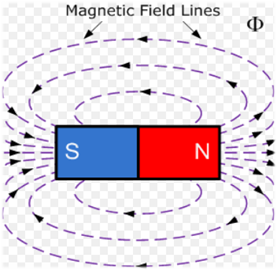Question 2.

See fig. Q-2, magnetic lines are shown. What is the direction of the current flowing through the wire?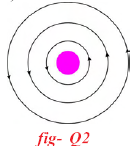According to the Right-Hand Thumb Rule. If we curl our fingers in the direction of the magnetic field lines than the thumb gives the direction of the flow of current.

In the above figure, the direction of magnetic field is anticlockwise Thus by curling our fingers in the anticlockwise direction the thumb points upwards. Therefore, the direction of the current is upwards. The figure below illustrates the same.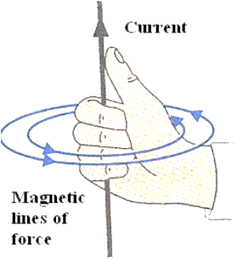Question 3.

A bar magnet with the North Pole facing towards a coil moves as shown in fig. Q-3. What happens to the magnetic flux passing through the coil?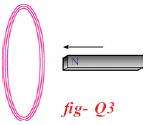Pushing the North Pole of the magnet towards a coil induces the current in the coil such that forward motion of the North Pole is opposed.

In order to oppose the motion the face of the coil opposite to the North Pole should also behave like a north pole.

Thus the current is induced in the anticlockwise direction. The figure below illustrates the generated induced current.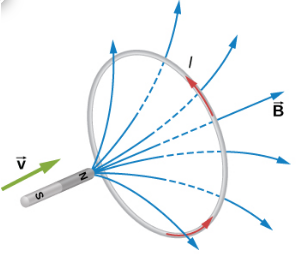Here B = Magnetic field;

V = velocity of magnet;

I = induced current.

Question 4.

A coil is kept perpendicular to the page. At P, current flows into the page and at Q it comes out of the page as shown in figure Q-4. What is the direction of magnetic field due to the coil?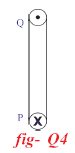As the current is flowing into the page at P and is coming out of the page at Q. Thus the direction of current is anticlockwise.

Therefore direction of the magnetic field is determined by Right-Hand Thumb Rule.If we curl our fingers in the anticlockwise direction than the thumb points outwards which is the direction of the flow of current.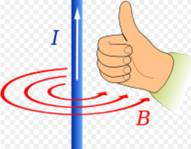Question 5.

The direction of current in a coil is shown in fig.Q.5. What type of magnetic pole as formed at the face that has a flow of current as shown in fig.Q-5?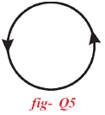In the figure given the direction of the current is in the anticlockwise direction. Therefore the North pole is formed at the face of the coil we are viewing.

The Figure below illustrates the same.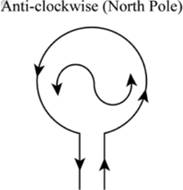Question 6.

Why does the picture appear distorted when a bar magnet is brought close to the screen of a television? Explain

Pictures on the Television screen is due to the motion of the electrons reaching the screen. Due to the fact that magnetic field exerts a force on moving charges motion of the electrons are affected by the magnetic field due to the bar magnet.

Therefore the picture appears distorted when a bar magnet is brought close to the screen of a television. And when the bar magnet is moved away from the screen the picture is clear.

Question 7.

Symbol 'X' indicates the direction of the magnetic field into the page. A straight long wire carrying current along its length is kept perpendicular to the magnetic field. What is the magnitude of force experience by the wire? In what direction does it act?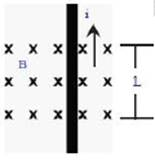Since the electric current is due to the charges which are in motion. Hence they must have a velocity let this be the drift velocity ‘V’.

Q = Total charge present on the conductor;

B = Magnetic field;

Magnetic force on the current carrying conductor is given by;

F = BQV. …(1)

Time is taken by the charge to cross the field =‘t’

∵ Velocity =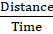⇒ V =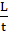Putting V in (1)

F =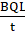F = BiL (∵ I = current =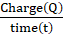).

Direction Of Force: By Right-Hand rule

1.) Thumb points in the direction of current (i).

2.) Finger points towards the direction of magnetic field (B).

3.) The thumb gives the direction of the current.

The figure below illustrates the same.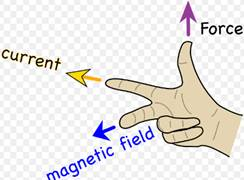The magnetic field is going inside the paper. Therefore the direction of force is towards left side.

Question 8.

Explain the working of an electric motor with a neat diagram

In the electric motors the electrical energy is converted into the mechanical energy.

Principle Of Electric Motor: Electric motor works on the principle that when a rectangular coil is placed in a magnetic field and current is passed through it. A force acts on the coil which rotates it continuously.

Working Of Electric Motor:

● Magnetic field is produced around the coil by passing the current into the rectangular coil.

● The magnetic field of the horse shoe magnet interacts with the magnetic field of the coil and causes the coil to rotate.

● When the ABCD is in the horizontal direction the current from the battery enters the coil through brushes B1 and leaves through brushes B2.

● Therefore the direction of current is from A to B and C to D.

● Force on side AB is in a downward direction and on side CD is in an upward direction. Thus the coil rotates in an anticlockwise direction.

● The reversing of current in the coil is repeated after every half rotation due to which the coil continues to rotate as long as current from the batter is passed through it.

The figure below illustrates the working of the electric motor.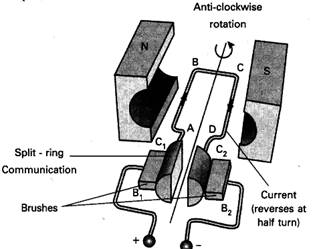Question 9.

Derive Faraday's law of induction from law of conservation of energy.

We know that Force applied on a wire in magnetic field is given by

F = BIL

Where;

B = Magnetic field;

I = Current in the wire;

L = Length of the wire;

Also, Work = Force × Distance;

⇒ W = FS.

⇒ W = BILS. (1) (∵ F = BIL).

When the wire moves in the magnetic field the decrease in flux (Φ) is

ΔΦ = BA

⇒ ΔΦ = BLS (2) (∵ Area = Length(L) x Breadth(S) )

From (1) W = BILS

W = (BLS)I.

From (2) ΔΦ = BLS

⇒ W = (ΔΦ)I .

Dividing Both sides by Δt.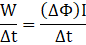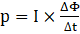(∵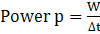)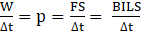(3)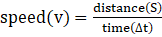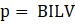( From (3) ).

Also Electrical Power (p) = E x I (4)

Where I = current;

E=EMF( Electromagnetic Force);

The Electrical power generated is used as the mechanical energy to move the wire. (Law of energy conservation)

⇒ EI = BILV (From (3) and (4) )

⇒ E = BLV.

∴ Induced EMF is BLV.

Question 10.

The value of magnetic field induction which is uniform is 2T. What is the flux passing through a surface of area 1.5m2 perpendicular to the field?

The Flux (Φ) is given by;

Φ = B x A .

B = Magnetic Field Strength

A = Surface area;

In our question B = 2T; A= 1.5m2

∴ Flux = 2 x 1.5;

Flux = 3.

Flux = 3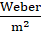.

Question 11.

A force of 8N acts on a rectangular conductor 20cm long placed perpendicular to a magnetic field. Determine the magnetic field induction if the current in the conductor is 40A.

We know that Force applied on a wire in a magnetic field is given by

F = BIL

Where;

B = Magnetic field;

I = Current in the wire;

L = Length of the wire;

In our question B = ?;

I = 40A;

L = 20cm = 0.2m (1m = 100cm);

F = 8N;

⇒ 8 = B x 40 x 0.2;

⇒ 8 = B x 8;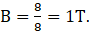Magnetic field induction is 1 Tesla

Question 12.

Explain with help of two activities that current carrying wire produces a magnetic field.

Activity 1:

Method:

i. Take a wooden sheet and fix two sticks in it .

ii. Join the sticks with the help of copper wire.

iii. Take the battery and key and connect them in series with the copper wire

iv. Place the compass below the copper wire

v. Press the key so that current flows in the circuit.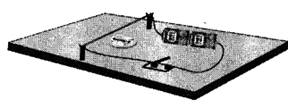Observation: The needle of the compass gets deflected.

Explanation: The current carrying copper wire produces the magnetic field which interacts with the magnet in the compass and deflects the needle of the compass.

Activity 2:

Method:

i. Take a wooden plank and make a hole in it .

ii. Place the plank on the table and place stand on it.

iii. Pass the copper wire through the hole in the plank and connect two ends of the copper wire with battery and switch

iv. Place compass needle around the hole and pass the current in the wire by pressing the switch.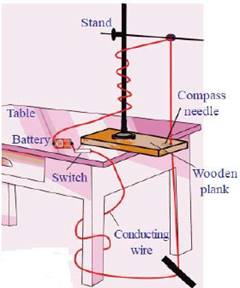Observation: The needle of the of the compass gets deflected.

Explanation: The current carrying copper wire produces the magnetic field which exerts magnetic force on the needle of the compass and it gets deflected.

Question 13.

How do you verify experimentally that the current carrying conductor experiences a force when it is kept in magnetic field?

Method:

i. Take a wooden plank and make two holes in it.

ii. Fix the two wooden sticks into the holes.

iii. Pass the copper wire through the splits of the wooden sticks

iv. Connect the wire to a 4V battery and close the switch to pass the current through the wire

v. Bring the horse shoe magnet near the wire.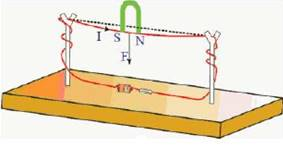Observation: The force is experienced on the wire And on reversing the polarities of the magnet the direction of the force get reversed.

Result and Explanation: The Magnetic field of the horse shoe magnet overlaps with the magnetic field produce by a current carrying wire. Thus the non-uniform magnetic field is created around the wire( shown in the figure below). Therefore the wire tries to move to the weaker region of the magnetic field.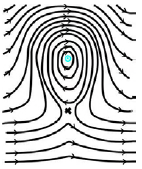Question 14.

Explain Faraday's law of induction with the help of activity.

i. Whenever there is the continuous change in the magnetic flux linked with the closed coil the current is generated in the coil.

ii. The current generated is called the induced current and the phenomenon is called as the Electromagnetic Induction.

Activity To Demonstrate Faraday’s Law: Connect the terminals of the coil to the sensitive galvanometer (Galvanometer is a device used to detect the current in the circuit ) as shown in the figure.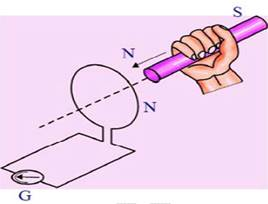Initially we will not see any deflection in the needle of the Galvanometer because there is no current in the circuit.

Bring the Bar Magnet near the coil and move it towards the coil and away from the coil.

Observation:

● When the bar magnet is move towards the coil with its north pole facing the coil The needle of the galvanometer gets deflected.

● The needle does not gets deflected when the bar magnet is at rest

● When the bar magnet is moved away from the coil the needle gets deflected in the opposite direction.

Result and Explanation:

On Moving the Bar magnet towards the coil and away from the coil the magnetic flux( The magnetic field lines) passing through the coil changes and this change of flux induces the current in the coil. This is the faraday’s law.

Question 15.

Explain the working of AC electronic generator with a neat diagram.

Definition: AC generator is the device which converts the mechanical energy into the electrical energy.

Principle: It works on the Faraday’s law of the Electromagnetic Induction which says that whenever a conductor is placed in a varying magnetic field, EMF is induced and this induced EMF is equal to the rate of change of flux.

Working:

i. A rectangular coil is held between the poles of two curved permanent magnets.

ii. As the coil rotates the Magnetic flux(due to permanent magnets) passing through the coil changes.

iii. Due to change in the magnetic flux, the induced current is generated in the coil.

iv. Thus converting the Mechanical Energy (Rotation of Coil) into the electrical energy(currently produced in the coil.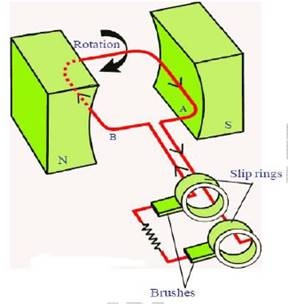Question 16.

Explain the working of DC generator with a neat diagram.

Definition: DC generator is the device which converts the mechanical energy into the electrical energy. The difference between AC and DC generator is that DC generator has split ring commutator instead of slip ring commutator present in AC generator.

Principle: It works on the Faraday’s law of the Electromagnetic Induction which says that whenever a conductor is placed in a varying magnetic field, EMF is induced and this induced EMF is equal to the rate of change of flux.

Working:

● A rectangular coil is held between the poles of two curved permanent magnets.

● As the coil rotates the Magnetic flux(due to permanent magnets) passing through the coil changes.

● Due to change in the magnetic flux the induced current is generated in the coil.

● When the coil is in vertical position the induced current generated during first half rotation rises from 0 to maximum and falls to zero again

● As the coil moves further the ends of coil goes to other slip rings.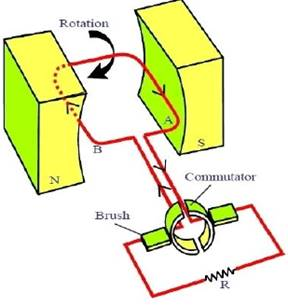Question 17.

Rajkumar said to you that the magnetic field lines are open and they start at North Pole of bar magnet and end at south pule. What questions do you ask Rajkumar to commit him by saying "field lines are closed”

The following questions I will ask to Raj Kumar in order to commit that field lines are closed.

1.) If magnetic field lines are starting from the North Pole and Ending at the South Pole than where they do go from the South Pole?

2.) Are Magnetic Field lines passing through the magnet?

3.) Why the magnetic field is experienced around the magnet?

4.) Will the Law Of Conservation of Energy be obeyed if the Magnetic Field Lines are open?

Question 18.

As shown in figure Q. 18 both coil and bar magnet moves in the same direction. Your friend is arguing that there is no change in flux. Do you agree with his statement? If not what doubts do you have? Frame questions about the doubts you have regarding change in flux.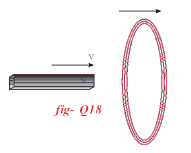No, I don’t agree with my friend. There will be the change in the flux. I will put following questions

1.) Do coil and the Magnet move with the same speed?

If their speed is same than their will be no relative motion between them and no flux will change

2.) How can I get the induced EMF without change in the flux?

3.) What are the various factors affecting the magnetic flux?

Question 19.

What experiment do you suggest to understand Faraday's law? What items are required? What suggestions do you give to get good results of the experiment? Give precautions also.

Aim: To study the Faraday’s Law.

Materials Required: A coil of copper wire; Bar Magnet; Galvanometer (For detecting the current in the circuit).

Procedure:

i. Connect the terminals of the coil to the sensitive galvanometer as shown in the figure.

ii. Keep the Bar Magnet stationary and note the observation.

iii. Push the bar magnet towards the coil with it’s North pole facing the coil and note the observation

iv. Move away the bar magnet from the coil and again note the observation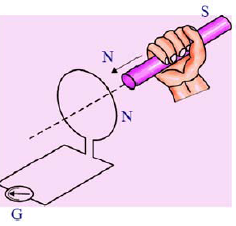Observation:

i. When the bar magnet is stationary no deflection is produced I the needle of the galvanometer.

ii. When the Bar Magnet is moved towards the coil the needle of the galvanometer gets deflected.

iii. When the Bar Magnet is moved away from the coil the needle of the galvanometer gets deflected in the opposite direction.

Result and Explanation:

i. On Moving the Bar magnet towards the coil and away from the coil the magnetic flux passing through the coil changes and this change of flux induces the current in the coil. This is the faraday’s law.

Precautions:

1. The coil should be kept on an insulating surface.

2. The center of the galvanometer must be at zero

3. The Bar Magnet should be of good strength.

Question 20.

How can you verify that a current carrying wire produces a magnetic field with the help of an experiment?

Aim: To verify that a current carrying wire produces a magnetic field

Procedure:

1. Take a wooden sheet and fix two sticks in it.

2. Join the sticks with the help of copper wire.

3. Take the battery and key and connect them in series with the copper wire

4. Place the compass below the copper wire

5. Press the key so that current flows in the circuit.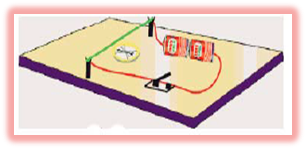Observation: The needle of the compass gets deflected.

Explanation: The current carrying copper wire produces the magnetic field which interacts with the magnet in the compass and deflects the needle of the compass.

Question 21.

1. Transformer Works on the principle of Electro Magnetic Induction (By faraday) which is used to transfer the current.

2. A Dynamo or DC generator which produces direct current works on the Faraday’s Law.

3. The current induced in the coil due to change in the magnetic flux.

4. The AC generator which produces alternating current also works on the Faraday’s law.

Question 22.

Collect the information about material required and procedure of making a simple electric motor from internal and make a simple motor on your own.

Aim: Preparation of the Electric motor.

Materials Required: A copper wire 20 cm long; 2V Battery; Iron nails; Strong Magnet; Paper clips.

Procedure:

1. Attach the Magnet to the head of the iron nail and paper clip to the other end of the magnet.

2. Attach the other end of the nail to the positive terminal of the battery.

3. Now connect the negative terminal of the battery and the head of the iron nail through the wire.

4. We will observe that the paper clip rotates and our simple motor is ready.

Question 23.

Collect information of experiments done by Faraday.

Faraday had conducted several experiments in the field of Physics some of them are as follows

1. Faraday's ice pail experiment: Demonstrated that electric charge resides on the exterior of the charged conductor.

2. Dynamo in Electromagnetic induction: Used for generating the current.

3. Homopolar motor in magnetism: A direct current electric motor.

4. Magnetic Deviation.

Question 24.

Draw a neat diagram of electric motor. Name the parts.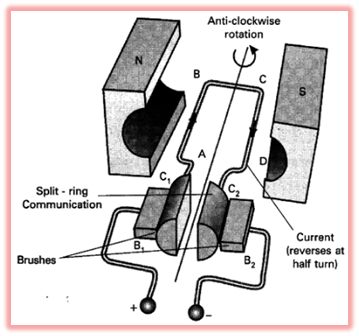ABCD is the rectangular coil which rotates.

‘N’ and ‘S’ are the two curved magnets.

‘B1’ and ‘B2’ are the brushes.

Commutator reverses the direction of the current.

Question 25.

Draw a neat diagram of an AC generator.